Project showcase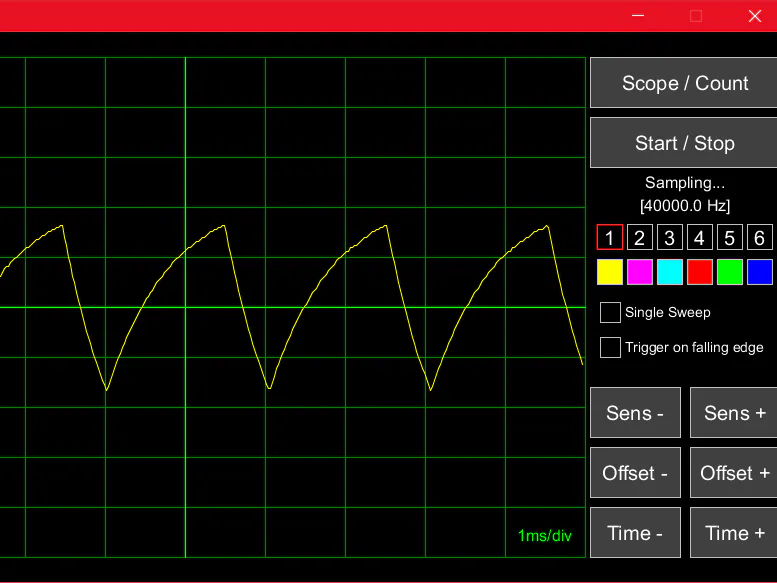# Arduino UNO Library for a Sampling Scope & Counter © LGPL

Easily include oscilloscope and frequency counter diagnostics into your own project.

• 6,724 views
• 29 respects

## Components and suppliesArduino UNO
×1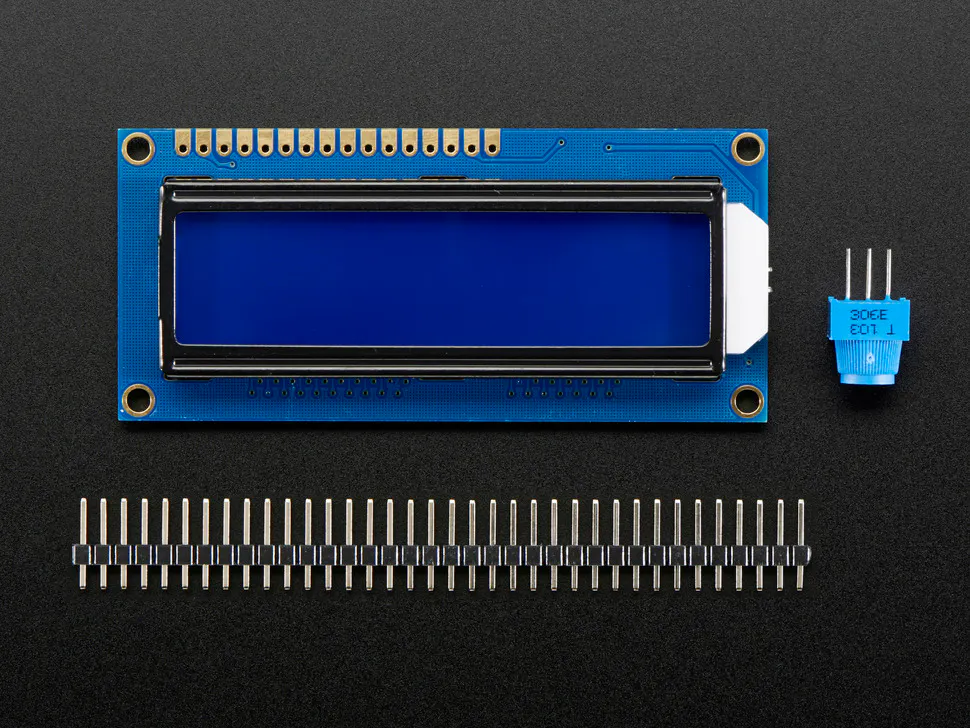Adafruit Standard LCD - 16x2 White on Blue
×1Resistor 1k ohm
×1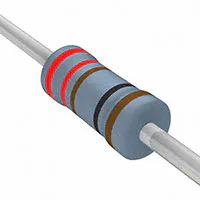Resistor 221 ohm
×4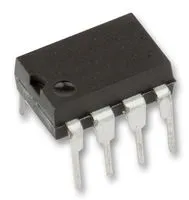555 Timers
×1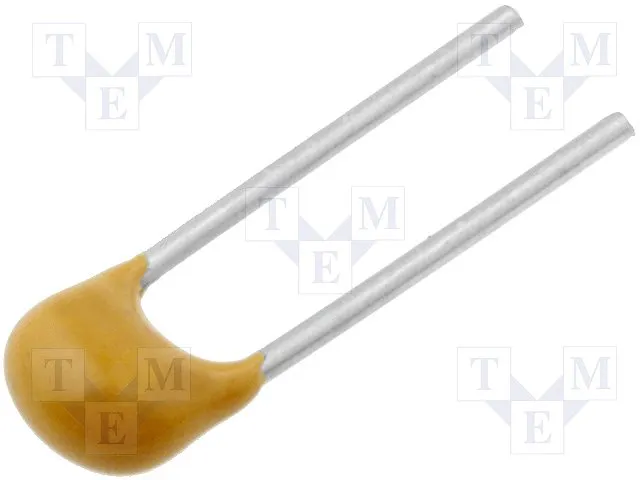Capacitor 100 nF
×2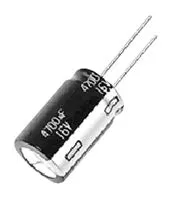Capacitor 1 µF
×1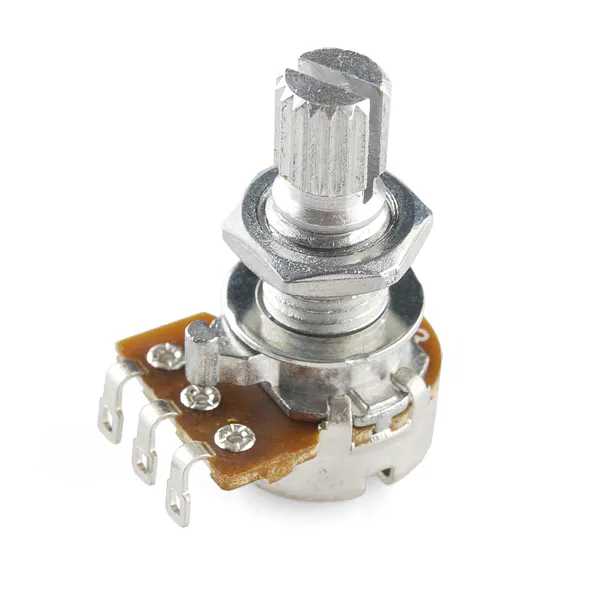Rotary potentiometer (generic)
×15 mm LED: Red
×2
 Resistor 470 kOhm
×1

After building my sampling scope and frequency counter, I figured it would be neat to be able to include these functions in a new project. That would make it easier to debug it, without the need of a second Arduino (since I only have one). This resulted in the library ScopeOne (for Arduino UNO) that you can easily include in your project. In the minimum configuration, the scope only uses two ports: a trigger input (port 2 or 3) and a signal input (one of A0-A5). Of course, you are still able to use all six channels of the original design if A0-A5 are not in use by your project.

### Improvements

• Slightly higher sampling frequency
• Reduced memory use
• Configurable input pins (a selection of A0-A5, trigger on pin 2 or 3)
• Added the use of the PC keyboard to communicate with Arduino

### How to use the library

First install the library. To do this, create a new folder "ScopeOne" in your "Arduino/libraries" folder (this is where the Ardiuno program is stored on your hard disk). Copy the library.properties and keyword.txt files to this folder. Then create a subfolder "src". Copy the ScopeOne.h and ScopeOne.cpp files to the src folder.

See the Arduino code files Oscilloscope.ino to see how to use the library. I've also added ScopeLCD.ino with an example of how to combine a project with ScopeOne.

### An example

The components listed for this projects are only needed to build the full example that uses an LCD, a wave generator circuit and a few LEDs. The scope itself can operate with the Arduino only. The example project also uses the Arduino LCD library. The project writes the operation mode to the display (Scope or Counter) as well as the first input channel pin and the number of channels. You may want to try and change the configuration to use fewer channels by altering the "ScopeOne::install();" statement into something like e.g. "ScopeOne::install(A2, 2);" and see what happens.

The number in the top right of the display is the contrast level of the LCD (analog output on pin 6), which can be controlled by '+' and '-' on the PC keyboard. The backlight of the display can be switched on and off using the '.' key.

The 8 kHz PWM signal on pin 6 can be viewed on Channel 6.

Pin 12 controls an LED, which is programmed to blink. The on/off cycles can be views on the scope (Channel 2) with a slow time base (1 sec/div).

The signals from the wave generator are on Channels 1, 3 and 4. The RC charging cycle is on Channel 1.

You may want to add some code to handle other keys pressed on the PC keyboard other than '-', '+' and '.' to, for example, change the blinking behaviour of the LED on pin 12.

Note that the ScopeOne library uses timers and interrupts that interfere with functions like delay(), millis() and microseconds(). These will not work in combination with the library. The Serial connection is opened automatically by ScopeOne at 115200 baud speed.

## Code

##### Oscilloscope.pdeProcessing
The code for the GUI of the oscilloscope running on your PC in the Processing IDE.
```/*
** Oscilloscope V2.0.1
*/
static boolean Debug = false;

import processing.serial.*;

boolean scope = false;

static final String SerialPort ="COM3";            // Change this to match your Arduino port

//static final String FontType = "System";

static final float fclk = 16e6;                    // Arduinos clock frequency

/* Commands to the Arduino board */
static final char Reset = 'X';
static final char ScopeMode = 'Y';
static final char CounterMode = 'Z';
static final char Channel1 = 'A';
static final char ChannelMax = 'F';
static final char TrigRising = 'w';
static final char TrigFalling = 'x';
static final char ContSweep = 'y';
static final char SingleSweep = 'z';
static final char TimeBaseMin = 'a';
static final char TimeBaseMax = 't';
static final char CounterBaseMin = 'G';
static final char CounterBaseMax = 'U';

/* Text definitions */
static final String Sampling = "Sampling...";
static final String SampleFreqFmt = "[%1.1f Hz]";
static final String FreqFmt = "%1.2f Hz";
static final String PeriodFmt = "T = %dx (/%d)";

/* End markers when switching to scope */
static final int EndMarkers = 3;

/* Trace definitions */
static final int MaxSample = 500;
static final int SampleSize = 8;
static final int SampleMax = (1 << SampleSize) - 1;
static final int Channels = ChannelMax - Channel1 + 1;

/* Screen size */
static final int MaxX = 1000;
static final int MaxY = 550;
/* Trace dimensions */
static final int Width = 800;
static final int Height = 500;

/* Time base parameters class */
class TimebaseSet
{
TimebaseSet(int f, float pwr, int s, float st)
{
factor = f;
p10 = pwr;
samples = s;
sampleTime = st;
}

int factor;
float p10;
int samples;
float sampleTime;
};

/* Class to execute a button action, used in conjunction with Button class */
abstract class ButtonAction
{
public abstract void execute();

public void setButton(Button b)
{
_button = b;
}
protected Button _button;
};

/* Class to represent a button with an associated action */
public class Button
{
public Button(int centerx, int centery, int w, int h, String name, long col, ButtonAction action)
{
_cx = centerx;
_cy = centery;
_buttonWidth = w;
_buttonHeight = h;
text = name;
red = (int) (col >> 16);
green = (int) ((col >> 8) & 0xFF);
blue = (int) (col & 0xFF);
_action = action;
if (_action != null)
{
_action.setButton(this);
}
}

void enable(boolean on)
{
enabled = on;
}

/* Shows the button on the screen */
public void draw()
{
if (enabled)
{
rectMode(CENTER);
fill(red, green, blue);
stroke(192, 192, 192);
rect(_cx, _cy, _buttonWidth, _buttonHeight);
textSize(20);
fill(255, 255, 255);
textAlign(CENTER, CENTER);
text(text, _cx, _cy - 3);
}
}

/* Checks if the button was clicked, executes the action if so */
public void isClicked(int x, int y)
{
int bw = _buttonWidth / 2;
int bh = _buttonHeight / 2;

boolean result = enabled && ((x >= _cx - bw && x <= _cx + bw && y >= _cy - bh && y <= _cy + bh));

if (Debug && result)
{
println(text + " clicked!");
}
if (result)
{
_action.execute();
}
}

protected int _cx;
protected int _cy;
protected int _buttonWidth;
protected int _buttonHeight;
public int red;
public int green;
public int blue;
public String text;
protected ButtonAction _action;
protected boolean enabled = true;
};

/* Class for check box buttons */
class CheckButton extends Button
{
class Toggle extends ButtonAction
{
public Toggle(ButtonAction action)
{
_taction = action;
}

public void execute()
{
if (Debug)
{
println(text + " toggled");
}
_state = !_state;
_taction.execute();
}

protected ButtonAction _taction;
};

public CheckButton(int centerx, int centery, int w, int h, String name, long col, ButtonAction action)
{
super(centerx, centery, w, h, name, col, null);

_action = new Toggle(action);
}

/* Shows the button on the screen */
public void draw()
{
rectMode(CENTER);
if (_state)
{
fill(255 - red, 255 - green, 255 - blue);
} else
{
fill(red, green, blue);
}
stroke(192, 192, 192);
rect(_cx, _cy, _buttonWidth, _buttonHeight);
textSize(14);
fill(255, 255, 255);
textAlign(LEFT, CENTER);
text(text, _cx + _buttonWidth / 2 + 5, _cy - 3);
}

/* Returns the current state of the check box */
public boolean getState()
{
return _state;
}

protected boolean _state = false;
};

/* Class for channel selection actions */
class ChAction extends ButtonAction
{
ChAction(int ch)
{
_ch = ch;
}

public void execute()
{
if (_button.red == 0 & _button.green == 0 && _button.blue == 0)
{
setChannel(_ch);
} else
{
showChannel(_ch);
}
}

protected int _ch;
};

int currentChannel = 0;

TimebaseSet[] timebase = {
//new TimebaseSet(1, 0.0001, 77, 0.000013), // 0
//new TimebaseSet(2, 0.0001, 154, 0.000013),
//new TimebaseSet(5, 0.0001, 384, 0.000013),
//new TimebaseSet(1, 0.0001, 71, 0.000014), // 0
//new TimebaseSet(2, 0.0001, 142, 0.000014),
//new TimebaseSet(5, 0.0001, 357, 0.000014),
//new TimebaseSet(1, 0.0001, 66, 0.000015), // 0
//new TimebaseSet(2, 0.0001, 133, 0.000015),
//new TimebaseSet(5, 0.0001, 333, 0.000015),
//new TimebaseSet(1, 0.0001, 62, 0.000016), // 0
//new TimebaseSet(2, 0.0001, 125, 0.000016),
//new TimebaseSet(5, 0.0001, 312, 0.000016),
new TimebaseSet(1, 0.0001, 50, 0.000020), // 0
new TimebaseSet(2, 0.0001, 100, 0.000020),
new TimebaseSet(5, 0.0001, 250, 0.000020),
//new TimebaseSet(1, 0.0001, 48, 0.000021), // 0
//new TimebaseSet(2, 0.0001, 95, 0.000021),
//new TimebaseSet(5, 0.0001, 238, 0.000021),
new TimebaseSet(1, 0.001, 400, 0.000025),
new TimebaseSet(2, 0.001, 400, 0.000050),
new TimebaseSet(5, 0.001, 500, 0.000100),
new TimebaseSet(1, 0.01, 500, 0.000200), // 6
new TimebaseSet(2, 0.01, 500, 0.000400),
new TimebaseSet(5, 0.01, 500, 0.001),
new TimebaseSet(1, 0.1, 500, 0.002),
new TimebaseSet(2, 0.1, 500, 0.004),
new TimebaseSet(5, 0.1, 500, 0.01),
new TimebaseSet(1, 1, 500, 0.02),
new TimebaseSet(2, 1, 500, 0.04),
new TimebaseSet(5, 1, 500, 0.1),
new TimebaseSet(1, 10, 500, 0.2),
new TimebaseSet(2, 10, 500, 0.4),
new TimebaseSet(5, 10, 500, 1.0),
new TimebaseSet(1, 100, 500, 2.0),
new TimebaseSet(2, 100, 500, 4.0)
};

int timebaseIndex = 7;
float timediv;
float sens;                     /* mV/div */

int samples;
int channelSamples[] = { 0, 0, 0, 0, 0, 0 };
float sampleTime;
float sample[][] = new float[Channels][MaxSample];

long  channelColor[] = { 0xFFFF00, 0xFF00FF, 0x00FFFF, 0xFF0000, 0x00FF00, 0x0000FF };
float  channelSampleTime[] = { 0, 0, 0, 0, 0, 0 };
boolean channelOn[] = { false, false, false, false, false, false };
boolean channelVisible[] = { false, false, false, false, false, false };

float periodCount;
float divider = 1024.0;
float count = 0.0;
float countDigit = 1.0;
float frequency = 0.0;
boolean countingInd = false;
char periodCountInd = CounterBaseMin;

Serial port;
PFont f;

int set = 0;
int index = 0;

int x0 = 0, x1 = Width;
int y0 = 25, y1 = Height + y0;
int divx = Width / 10;
int divy = Height / 10;

float scalex;
float scaley;
float xcenter = (x0 + x1) / 2;
float ycenter = (y0 + y1) / 2;
float offset[] = { 0, 0, 0, 0, 0, 0 };
int sensFact[] = { 5, 5, 5, 5, 5, 5 };
int sens10[] = { 100, 100, 100, 100, 100, 100 };

boolean measuring = false;
int flushingCountData = 0;
boolean getChannelCount = false;

ArrayList<Button> button = new ArrayList();
CheckButton sweepButton;
CheckButton triggerButton;

void scale()
{
scalex = Width / timediv / 10.0;
scaley = Height / (sens / 1000.0) / 10.0;
}

float plotX(float time)
{
return scalex * time;
}

float plotY(int channel, float voltage)
{
return y1 - scaley * (voltage + offset[channel]);
}

/* Switch between scope and frequency counter mode */
void toggleMode()
{
scope = !scope;
getChannelCount = scope;    // Wait for the number of channels reported by Arduino
flushingCountData = (scope ? EndMarkers : 0);
port.write(scope ? ScopeMode : CounterMode);
port.clear();
if (scope)
{
updateTimebase();
} else
{
scope = false;
updatePeriodCount();
}
}

/* Switch active channel for receiving samples */
void setChannel(int ch)
{
channelOn[currentChannel] = false;
currentChannel = ch;
for (int i = 0; i < MaxSample; i++)
{
sample[currentChannel][i] = 0;
}
channelSamples[currentChannel] = samples;
channelOn[currentChannel] = true;
channelVisible[currentChannel] = true;
sens = sensFact[currentChannel] * sens10[currentChannel];
port.write((char) (currentChannel + Channel1));  // Send channel switch command to Arduino
index = 0;
}

/* Toggle a channel's trace visibility */
void showChannel(int ch)
{
channelVisible[ch] = !channelVisible[ch];
}

/* Handle start/stop button press */
void startStop()
{
measuring = !measuring;
index = 0;
if (measuring && sweepButton.getState())
{
if (!Debug)
{
port.write(Reset);    // Send a reset command to Arduino
}
}
}

/* Toggle sweep mode between single and continuous */
void setSweep()
{
if (!Debug)
{
port.write(sweepButton.getState() ? SingleSweep : ContSweep);
}
index = 0;
}

/* Toggle trigger mode between rising and falling edge */
void setTriggerMode()
{
if (!Debug)
{
port.write(triggerButton.getState() ? TrigFalling : TrigRising);
}
index = 0;
}

/* Increase sensitivity */
void sensUp()
{
if (sens > 10.0)
{
sensFact[currentChannel] /= 2;
if (sensFact[currentChannel] == 0)
{
sens10[currentChannel] /= 10;
sensFact[currentChannel] = 5;
}
}
}

/* Decrease sensitivity */
void sensDn()
{
if (sens < 5000.0)
{
sensFact[currentChannel] *= 2;
if (sensFact[currentChannel] == 4)
{
sensFact[currentChannel] = 5;
}
if (sensFact[currentChannel] >= 10)
{
sens10[currentChannel] *= 10;
sensFact[currentChannel] = 1;
}
}
}

/* Update time base based on the value of timebaseIndex */
void updateTimebase()
{
timediv = (float) timebase[timebaseIndex].factor * timebase[timebaseIndex].p10;
samples = timebase[timebaseIndex].samples;
sampleTime = timebase[timebaseIndex].sampleTime;
channelSamples[currentChannel] = samples;
scale();
if (!Debug)
{
port.write((char) (timebaseIndex + TimeBaseMin));    // Send command to Arduino
}
index = 0;
}

/* Increase time base (slower scan) */
void timeUp()
{
if (scope)
{
if (timebaseIndex < TimeBaseMax - TimeBaseMin)
{
timebaseIndex++;
updateTimebase();
}
} else
{
if (periodCountInd < CounterBaseMax)
{
periodCountInd++;
updatePeriodCount();
}
}
}

/* Decrease time base (faster scan) */
void timeDn()
{
if (scope)
{
if (timebaseIndex > 0)
{
timebaseIndex--;
updateTimebase();
}
} else
{
if (periodCountInd > CounterBaseMin)
{
periodCountInd--;
updatePeriodCount();
}
}
}

/* Update periods */
void updatePeriodCount()
{
periodCount = 1.0;
divider = 64.0;
int s = periodCountInd - CounterBaseMin;
int p = s / 3;
int d = 2 - (s % 3);
for (int div = 0; div < d; div++)
{
divider *= 4.0;
}
for (int per = 0; per < p; per++)
{
periodCount *= 10.0;
}
port.write(periodCountInd);
count = 0.0;
countDigit = 1.0;
}

/* Initiate */
void setup()
{
if (!Debug)
{
port = new Serial(this, SerialPort, 115200);
} else
{
// For testing simulate a sine wave
for (index = 0; index < MaxSample; index++)
{
sample[index] = 2.5*sin(2.0*PI*20.0*((float) (index * sampleTime / 1000.0)))+2.5;
}
}

// Screen
size(1000, 550);
frameRate(50);
background(0);
//f = createFont(FontType, 16);

// Define buttons
button.add(new Button(900, 50, 190, 50, "Scope / Count", 0x404040, new ButtonAction() {
public void execute() {
toggleMode();
}
}
));
button.add(new Button(900, 110, 190, 50, "Start / Stop", 0x404040, new ButtonAction() {
public void execute() {
startStop();
}
}
));
for (int ch = 0; ch < Channels; ch++)
{
button.add(new Button(825 + ch * 30, 205, 25, 25, "" + (char) ('1' + ch), 0, new ChAction(ch)));
}
for (int ch = 0; ch < Channels; ch++)
{
button.add(new Button(825 + ch * 30, 240, 25, 25, "", channelColor[ch], new ChAction(ch)));
}
button.add(sweepButton = new CheckButton(825, 280, 20, 20, "Single Sweep", 0x000000, new ButtonAction() {
public void execute() {
setSweep();
}
}
));
button.add(triggerButton = new CheckButton(825, 315, 20, 20, "Trigger on falling edge", 0x000000, new ButtonAction() {
public void execute() {
setTriggerMode();
}
}
));
button.add(new Button(850, 380, 90, 50, "Sens -", 0x404040, new ButtonAction() {
public void execute() {
sensDn();
}
}
));
button.add(new Button(950, 380, 90, 50, "Sens +", 0x404040, new ButtonAction() {
public void execute() {
sensUp();
}
}
));
button.add(new Button(850, 440, 90, 50, "Offset -", 0x404040, new ButtonAction() {
public void execute()
{
if (offset[currentChannel] > -15.0)
{
offset[currentChannel] -= 0.5;
}
}
}
));
button.add(new Button(950, 440, 90, 50, "Offset +", 0x404040, new ButtonAction() {
public void execute()
{
if (offset[currentChannel] < 15.0)
{
offset[currentChannel] += 0.5;
}
}
}
));
button.add(new Button(850, 500, 90, 50, "Time -", 0x404040, new ButtonAction() {
public void execute() {
timeDn();
}
}
));
button.add(new Button(950, 500, 90, 50, "Time +", 0x404040, new ButtonAction() {
public void execute() {
timeUp();
}
}
));

// Set initial configuration of the scope (matches Arduino defaults)
updateTimebase();
updatePeriodCount();
setChannel(currentChannel);
startStop();
}

void draw()
{
clear();

if (measuring & scope)
{
textSize(16);
fill(255, 255, 255);
text(Sampling, 900, 147);
text(String.format(SampleFreqFmt, 1.0 / sampleTime), 900, 170);
}

/* Gridlines */
stroke(0, 128, 0);

/* Vertical */
for (int x = 0; x <= x1; x += divx)
{
line(x, y1, x, y0);
}
/* Horizontal */
for (int y = y0; y <= y1; y += divy)
{
line(x0, y, x1, y);
}

/* Emphasize horizontal and vertical center lines */
stroke(0, 255, 0);
line(xcenter, y0, xcenter, y1);
line(x0, ycenter, x1, ycenter);

/* Show all buttons */
for (int b = 0; b < button.size(); b++)
{
button.get(b).draw();
}

/* Show active channel */
stroke(255, 0, 0);
noFill();
rect(825 + currentChannel * 30, 205, 25, 25);

/* Scaling info text */
textSize(16);
fill(255, 255, 255);
textAlign(LEFT, CENTER);
//textFont(f);
fill(0, 255, 0);
sens = sensFact[currentChannel] * sens10[currentChannel];
text(String.format("%d%cV/div", (int) (sens > 999 ? sens / 1000 : sens),
sens < 1000.0 ? 'm' : '\0'), x0 + 4, y0 + divy / 4);
text(String.format("Offset: %+1.1fV", offset[currentChannel]), x0 + 4, y0 + 3 * divy / 4);

int tb = (int) (timediv);
char unit = ' ';

if (timediv < 0.001)
{
tb = (int) (timediv * 1000000.0 + 0.5);
unit = 'u';
} else if (timediv < 1.0)
{
tb = (int) (timediv * 1000.0 + 0.5);
unit = 'm';
}

textAlign(CENTER, CENTER);
text(String.format("%d%cs/div", tb, unit), x0 + 19 * divx / 2, y0 + 19 * divy / 2);

if (scope)
{
/* Display the sample traces */

for (int ch = 0; ch < Channels; ch++)
{
if (channelVisible[ch])
{
sens = sensFact[ch] * sens10[ch];
scale();
float prevx = plotX(0.0);
float prevy = plotY(ch, sample[ch]);
long c = channelColor[ch];

prevy = max(prevy, y0);
prevy = min(prevy, y1);

stroke(c >> 16, (c >> 8) & 0xFF, c & 0xFF);

for (int i = 1; i < channelSamples[currentChannel] && prevx < x1; i++)
{
float x = plotX(i * sampleTime);
float y = plotY(ch, sample[ch][i]);
if (y <= y1 && y >= y0)
{
line(prevx, prevy, x, y);
prevx = x;
prevy = y;
}
}
}
}
} else
{
rectMode(CENTER);
stroke(255, 255, 255);
fill(0, 0, 0);
rect((x0 + x1) / 2, ycenter, 4 * divx, 2 * divy);

if (countingInd)
{
fill(255, 0, 0);
rect(xcenter - 7 * divx / 4, ycenter - 3 * divy / 4, 20, 20);
}

textAlign(CENTER, CENTER);
textSize(30);
fill(255, 255, 255);
text(String.format(FreqFmt, frequency), xcenter, ycenter - divy / 4);
textSize(18);
text(String.format(PeriodFmt, (int) periodCount, (int) divider), xcenter, ycenter + divy / 2);
}
sens = sensFact[currentChannel] * sens10[currentChannel];
}

/* Handle button clicks */
void mouseClicked()
{
int mx = mouseX;
int my = mouseY;

for (int b = 0; b < button.size(); b++)
{
button.get(b).isClicked(mx, my);
}
}

void keyPressed()
{
if (!Character.isLetter(key))
{
port.write(key);
}
}

/* Handle incoming sample data from Arduino */
void serialEvent(Serial port)
{
int s;
float v;

try
{
if (port.available() != 0)
{
v = s;
if (s == 0xFF)    // End-of-sweep indicator
{
if (scope)
{
if (flushingCountData > 0)
{
//println("s==0xFF");
//println ("flushCount=", flushingCountData);
flushingCountData--;
}
index = 0;
} else
{
if (countDigit >= 4294967296.0)
{
if (count > 0)
{
frequency = fclk * periodCount / (count * divider) ;
}
count = 0.0;
countDigit = 1;
countingInd = !countingInd;
} else
{
count += (v * countDigit);
countDigit *= 256;
}
}
} else
{
if (scope)
{
if (getChannelCount)
{
//println ("flushCount=", flushingCountData);
if (flushingCountData == 0)            // If 3 consecutive 0xFF found, number of channels follows
{
getChannelCount = false;
//println ("s=", s);
s -= '0';
//println (s, "Channels");
for (int i = 0; i < 6; i++)
{
button.get(2 + i).enable(i < s);
button.get(8 + i).enable(i < s);
}
} else
{
//println("s==", s);
flushingCountData = EndMarkers;
}
}
if (measuring)
{
sample[currentChannel][index++] = map(s, 0.0, 255.0, 0.0, 5.0);
if (index >= channelSamples[currentChannel])
{
index = 0;
if (sweepButton.getState())
{
measuring = false;
}
}
}
} else
{
count += (v * countDigit);
countDigit *= 256;
}
}
}
}

catch(RuntimeException e)
{
e.printStackTrace();
}
}
```
##### Oscilloscope.inoArduino
The minimum code to create an oscilloscope on your Arduino using the ScopeOne library
```/*
Oscilloscope using the ScopeOne library
*/

// Uncomment the next line to use pin 3 instead of pin 2 as trigger input
//#define TriggerPin 3

#include <ScopeOne.h>

void setup()
{
ScopeOne::install();

// Other ways of configuring the scope:
// ScopeOne::install(A2);     // Uses A2-A5 as inputs
// ScopeOne::install(A3, 2);  // Uses A3 and A4 as inputs (2 channels)
}

void loop()
{
char ch = ScopeOne::getCommand();

// ch will be 0 if no command was received
// ch will be ScopeOneCommand if a scope command was received and handled
// ch will be the character typed on the keyboard of your PC (not a letter)
}
```
##### ScopeLCD.inoArduino
An example project that uses the ScopeOne library together with an LCD, an NE555 based wave generator and a blinking LED
```/*
* ScopeLCD demonstrates the use of the ScopeOne library used in
* combination with an LCD display
*/

#include <ScopeOne.h>
#include <LiquidCrystal.h>

#define PwmFreq65k 0x1
#define PwmFreq7k 0x2
#define PwmFreq976 0x3
#define PwmFreq244 0x4
#define PwmFreq061 0x5

// Associate LCD interface pins
const int rs = 3, rw = 4, en = 5, d4 = 8, d5 = 9, d6 = 10, d7 = 11;
// Associate display contrast and backlight control pins
const int contrast = 6, backlight = 7;
// An LED to play with
const int Led = 12;

LiquidCrystal lcd(rs, en, d4, d5, d6, d7);

// The actual contrast value
int co = 70;

void setup()
{
pinMode(rw, OUTPUT);
digitalWrite(rw, LOW); // Not used by LCD library, set to write

pinMode(contrast, OUTPUT);
pinMode(backlight, OUTPUT);
analogWrite(contrast, co);
digitalWrite(backlight, HIGH);

pinMode(Led, OUTPUT);

// Set up the LCD: 16 characters, 2 rows
lcd.begin(16, 2);

// Install the scope
ScopeOne::install();

TCCR0B = (TCCR0B & 0xF8) | PwmFreq7k;
//  TCCR0B = (TCCR0B & 0xF8) | PwmFreq976;           // Set pin 5/6 PWM frequency

// Print the scope configuration to the LCD
lcd.setCursor(0, 0);
lcd.print(ScopeOne::theScope->isScopeMode() ? "Scope" : "Counter");
lcd.setCursor(0, 1);
lcd.print("Ch1=A");
lcd.print(ScopeOne::theScope->getChannel1() - A0);
lcd.print(", #ch=");
lcd.print(ScopeOne::theScope->getNrChannels());
}

void loop()
{
{
digitalWrite(Led, HIGH + LOW - digitalRead(Led));
}

switch (ScopeOne::getCommand())
{
case 0:
break;
case '-':       // Decrease contrast
if (co > 0)
{
co--;
analogWrite(contrast, co);
}
break;
case '+':       // Increase contrast
if (co < 255)
{
co++;
analogWrite(contrast, co);
}
break;
case '.':       // Toggle backlight
digitalWrite(backlight, HIGH + LOW - digitalRead(backlight));
break;
case Reset:     // Scope command received, update info
// Print a message to the LCD.
lcd.setCursor(0, 0);
lcd.print(ScopeOne::theScope->isScopeMode() ? "Scope  " : "Counter");
break;
default:
// Ignore other key strokes
break;
}

// Show the contrast value
lcd.setCursor(13, 0);
lcd.print(co);
lcd.print("  ");
}
```
##### library.propertiesProperties
ScopeOne library property file
```name=ScopeOne
version=1.0.0
author=Qixaz
maintainer=Qixaz (www.qixaz.com)
sentence=Digital sampling oscilloscope and frequency counter for diagnosing your circuits
paragraph=Library to use your Arduino One as a digital sampling scope and frequency counter. You can create the scope with just a few commands in your project code and diagnose your circuit.
category=Data Processing
url=http://www.qixaz.com
architectures=*
```
##### keywords.txtPlain text
Keyword file for the ScopeOne library
```#######################################
# Syntax Coloring Map For ScopeOne
#######################################

#######################################
# Class (KEYWORD1)
#######################################

ScopeOne	KEYWORD1	ScopeOneLibrary

#######################################
# Methods and Functions (KEYWORD2)
#######################################

# method names in ScopeOne

install		KEYWORD2
create		KEYWORD2
setup		KEYWORD2
isScopeMode	KEYWORD2
getChannel1	KEYWORD2
getNrChannels	KEYWORD2
getCommand	KEYWORD2

#######################################
# Constants (LITERAL1)
#######################################

DfltOnTime		LITERAL1
MinusPattern	LITERAL1
```
```#ifndef _Scope_h_
#define _Scope_h_

/*
1-6 channel oscilloscope

Time base:
100, 200, 500 us
1, 2, 5 ms
10, 20, 50 ms
100, 200, 500 ms
1, 2, 5 s
10, 20, 50 s

Counter time bases:
1 period, clock divider 1024
1 period, clock divider 256
1 period, clock divider 64
10 periods, clock divider 1024
...
10000 periods, clock divider 64
*/

#include <Arduino.h>

#define MaxChannels 6

// Commands to the Arduino board
#define Reset 'X'
#define ScopeMode 'Y'
#define CounterMode 'Z'
#define Channel1 'A'
#define ChannelMax 'F'
#define TrigRising 'w'
#define TrigFalling 'x'
#define ContSweep 'y'
#define SingleSweep 'z'
#define TimeBaseMin 'a'
#define TimeBaseMax 't'
#define CounterBaseMin 'G'
#define CounterBaseMax 'U'
#define InitialMode 'Z'

#define ScopeOneCommand 'X'

// The trigger default is pin 2, unless user has defined it otherwise
#ifndef TriggerPin
#define TriggerPin 2
#endif

#define MaxSamples 500

// Timer and interrupt settings
#define INTBIT B00000001
#define TRIGCLR B00000001
#define TRIGRISE B00000011
#define TIMERCTCA B00000000
#define TIMERCTCB B10001000
#define TIMERCNTA B00000000
#define TIMERCNTB B00000000
#define TIMERNOCLK B11111000
#define TIMERPS0001 B00000001
#define TIMERPS0256 B00000100
#define TIMERPS1024 B00000101

#define InitValue 123

class ScopeOne
{
public:
static ScopeOne* install(byte channel1 = A0, byte nrChannels = MaxChannels);
static ScopeOne* create(byte channel1 = A0, byte nrChannels = MaxChannels);
void setup();

static char getCommand();

bool isScopeMode();
byte getChannel1();
byte getNrChannels();

inline void trigger();
inline void getSample();

static ScopeOne* theScope;

protected:
// Constructor
ScopeOne(byte channel1, byte nrChannels);

// Command handler
char execute(char c);

// Scope functions
void setScope();
void scopeReset();
void setChannel(byte c);
void setSampleTime(byte c);
void setTriggerMode(byte c);
void setSweepMode(byte c);
void stopSweep();
void writeData();

// Counter functions
void setCounter();
void counterReset();
void setPeriods(byte c);
void writeCount(unsigned long cnt);

void init();

bool scope;
bool continuousSweep;
byte maxChannels;
byte firstChannel;
byte currentChannel;
char currentBase;

// Sample variables
int samples;
volatile byte sample[MaxSamples];
volatile int index;
volatile int writeIndex;

// Frequencey counter current ode variables
int periodCount;
volatile int periods;
volatile unsigned long count;

byte timerPrescaler;
byte intBit;
volatile byte counterDiv;
bool writeImmediate;

static byte _isInitialized;
};

#endif
```
##### ScopeOne.cppC/C++
ScopeOne C++ source code
```#include <ScopeOne.h>

ScopeOne* ScopeOne::theScope = NULL;
byte ScopeOne::_isInitialized = 0;

// Create ad set-up a scope
ScopeOne* ScopeOne::install(byte channel1, byte nrChannels)
{
create(channel1, nrChannels);
theScope->setup();
return theScope;
}

// Create a scope object
ScopeOne* ScopeOne::create(byte channel1, byte nrChannels)
{
if (_isInitialized != InitValue)
{
delete theScope;
}
theScope = new ScopeOne(channel1, nrChannels);
_isInitialized = InitValue;
return theScope;
}

// Constructor
ScopeOne::ScopeOne(byte channel1, byte nrChannels)
{
if (channel1 < A0 || channel1 > A5)
{
firstChannel = A0;
maxChannels = 1;
}
else
{
if (channel1 + nrChannels - 1 >= A5)
{
maxChannels = A5 - channel1 + 1;
}
else
{
maxChannels = nrChannels <= MaxChannels ? nrChannels : MaxChannels;
}
firstChannel = channel1;
}
scope = true;
intBit = (INTBIT << (TriggerPin == 2 ? 0 : 1));
currentChannel = 0;
counterDiv = TIMERPS1024;
}

byte ScopeOne::getChannel1()
{
return firstChannel;
}

byte ScopeOne::getNrChannels()
{
return maxChannels;
}

/* Return scope mode */
bool ScopeOne::isScopeMode()
{
return scope;
}

/* Initialize the Analog-Digital Converter */
{
}

{
sample[index++] = ADCH;                         // 8-bit sample size for speed
}

/* Handle the end of a sweep */
void ScopeOne::stopSweep()
{
TCCR1B &= TIMERNOCLK;                           // Set clock select to 0 (no clock)
index++;
writeData();                                    // Write sampled data to serial connection
if (continuousSweep)
{
scopeReset();                                 // Restart automatically in continuous sweep mode
}
}

/* Reset the scope for a new sweep */
void ScopeOne::scopeReset()
{
TCCR1B &= TIMERNOCLK;                           // Stop the timer by setting clock select to 0 (no clock)

Serial.print((char) 0xFF);                      // Mark end of sweep to console

index = 0;                                      // Reset sweep data
writeIndex = 0;

EIFR |= intBit;                                 // Reset trigger interrupt flag
EIMSK |= intBit;                                // Enable interrupts on trigger input

// Wait for trigger signal interrupt
}

/* Reset the frequency counter to start another measurement */
void ScopeOne::counterReset()
{
periods = 0;                                    // Reset counted periods
count = 0UL;                                    // Reset total timer counts
TCNT1 = 0;                                      // Reset timer
EIFR != intBit;
}

/* Set the sample time for the selected time base.
* The selection is done with a single character 'a'-'t'.
*/
void ScopeOne::setSampleTime(byte c)
{
unsigned int cnt;

switch (c)
{
case 0:
case 1:
case 2:
case 3:
break;
case 4:
break;
case 5:
break;
default:
break;
}

// Set #samples
switch (c)
{
case 0:
// samples = 77;
// samples = 71;
// samples = 66;
// samples = 62;
samples = 50;
// samples = 48;
break;
case 1:
// samples = 154;
// samples = 142;
// samples = 133;
// samples = 125;
samples = 100;
// samples = 95;
break;
case 2:
// samples = 384;
// samples = 357;
// samples = 333;
// samples = 312;
samples = 250;
// samples = 238;
break;
case 3:
case 4:
samples = 400;
break;
default:
samples = 500;
break;
}

// Set timer prescaler
timerPrescaler = (c <= 9 ? TIMERPS0001 : (c <= 17 ? TIMERPS0256 : TIMERPS1024));

// Set counter max value
switch (c)
{
case 0:
case 1:
case 2:
// cnt = 208;
// cnt = 224;
// cnt = 240;
// cnt = 256;
cnt = 320;
// cnt = 336;
break;
case 3:
case 4:
case 5:
case 6:
case 7:
cnt = 400 << (c - 3);
break;
case 8:
cnt = 16000;
break;
case 9:
cnt = 32000;
break;
case 10:
cnt = 250;
break;
case 11:
case 12:
case 13:
cnt = 625 << (c - 11);
break;
case 14:
case 15:
case 16:
cnt = 6250 << (c - 14);
break;
case 17:
case 19:
cnt = 62500;
break;
case 18:
cnt = 31250;
break;
}
OCR1A = cnt;

writeImmediate = (c >= 8);
}

/* Set trigger mode to falling or rising edge */
void ScopeOne::setTriggerMode(byte c)
{
if (c == 1)
{
EICRA &= ~(TRIGCLR << (TriggerPin == 2 ? 0 : 2)); // 1 is falling edge
}
else
{
EICRA |= TRIGRISE << (TriggerPin == 2 ? 0 : 2);	 // 0 is rising edge
}
}

/* Sweep mode (continuous or single) */
void ScopeOne::setSweepMode(byte c)
{
continuousSweep = (c == 0);                     // 'y' is continuous, 'z' is single
}

/* Set the channel 'A'-'F' */
void ScopeOne::setChannel(byte c)
{
if (c < maxChannels)
{
byte offset = firstChannel - A0;
currentChannel = c;
ADMUX |= ((offset + currentChannel) & 0x7);            // Switch the ADC multiplexer to the channel pin
}
}

/* Start oscilloscope mode */
void ScopeOne::setScope()
{
TCCR1B = 0;                                   // ... Stop counter
scope = true;

TCCR1A = TIMERCTCA;                           // Use Timer1 in 'match OCR' mode for sampling
TCCR1B = TIMERCTCB;                           // No clock, so no interrupts yet

Serial.print((char) 0xFF);					  // Mark sending of channel number
Serial.print((char) 0xFF);
Serial.print((char) (getNrChannels() + '0')); // Send channel number to PC

TIMSK1 |= (1 << OCIE1A);                      // Enable timer1 compare interrupts
execute(currentBase);                         // Set the time base to the last used
scopeReset();                                 // Restart scope
}

/* Start frequency counter mode */
void ScopeOne::setCounter()
{
scope = false;
periodCount = 1;
TCCR1A = TIMERCNTA;                           // Use Timer1 in normal mode for counting
TCCR1B = 0;                                   // Hold timer
TIMSK1 &= ~(1 << OCIE1A);                     // Disable timer1 compare interrupts
EIFR |= intBit;
EIMSK |= intBit;                              // Enable external interrupt
counterReset();                               // Restart frequency counter
}

/* Set the number of periods to count for determining frequency */
void ScopeOne::setPeriods(byte c)
{
int p = c / 3;                                  // Period count 1, 10, 100, 1000 or 10000
counterDiv = 5 - (c % 3);                       // Clock divider 64, 256 or 1024 for accuracy
periodCount = 1;
for (int per = 0; per < p; per++)
{
periodCount *= 10;
}
}

/* Handle command characters sent from the console */
char ScopeOne::execute(char c)
{
switch (c)
{
case Reset:
// Reset always happens at the end of execute
break;
case TrigRising:
case TrigFalling:
setTriggerMode(c - TrigRising);
break;
case ContSweep:
case SingleSweep:
setSweepMode(c - ContSweep);
break;
case CounterMode:
setCounter();
break;
case ScopeMode:
setScope();
break;
default:
if (c >= Channel1 && c <= ChannelMax)
{
setChannel(c - Channel1);
}
else if (c >= TimeBaseMin && c <= TimeBaseMax)
{
setSampleTime(c - TimeBaseMin);
// Store the time base as current
currentBase = c;
}
else if (c >= CounterBaseMin && c <= CounterBaseMax)
{
setPeriods(c - CounterBaseMin);
}
else
{
return c;								  // No scope command, pass on to user's program
}
}
if (scope)
{
scopeReset();
}
else
{
counterReset();
}
return Reset;									  // Indicates a scope command was processed
}

/* Send all available samples to the console */
void ScopeOne::writeData()
{
for (; writeIndex < index; writeIndex++)
{
Serial.print((char) sample[writeIndex]);
}
}

/* Writes the count value for the defined number of periods in 4 bytes, LSB first */
void ScopeOne::writeCount(unsigned long cnt)
{
unsigned long c = cnt;

for (int d = 0; d < 4; d++)
{
Serial.print((char) (c & 0xFF));
c >>= 8;
}
Serial.print((char) (0xFF));                    // Send all ones to mark end of transmission
}

/* Standard set-up */
void ScopeOne::setup()
{
byte pin = firstChannel;

Serial.begin(115200);                     	  // Fast serial connection

pinMode(TriggerPin, INPUT_PULLUP);            // The trigger input

for (int i = 0; i < maxChannels; i++, pin++)  // Channel inputs
{
pinMode(pin, INPUT);
}

TIMSK0 = 0;                                     // Disable other timer interrupts
TIMSK2 = 0;

// External interrupt for trigger signal
EIMSK &= ~intBit;                               // Disable trigger interrupt first;
EIFR |= intBit;                                 // Clear pending interrupts
EICRA = TRIGRISE << (TriggerPin == 2 ? 0 : 2);  // Start with rising edge

// Set the default controls
execute(TrigRising);                            // Rising edge trigger
execute(ContSweep);                             // Continuous sweep
execute(Channel1);                              // Channel A0
execute(TimeBaseMin + 7);                       // Time base at 10ms/div
execute(CounterBaseMin);                        // Counter time base at 1x/1024

execute(InitialMode);                           // Start in selected initial mode
}

char ScopeOne::getCommand()
{
if (Serial.available())                         // If a command was sent from the console, ...
{
return theScope->execute(Serial.read());      // ...handle it here
}
return 0;
}

inline void ScopeOne::trigger()
{
if (scope)
{
EIMSK &= ~intBit;
EIFR |= intBit;

TCNT1 = 0;                                    // Reset timer
TCCR1B |= timerPrescaler;                     // Start timer now
}
else
{
int c = TCNT1;

TCNT1 = 0;
TCCR1B = counterDiv;                          // Start counter
count += c;                                   // Add current timer to total count
periods++;                                    // Another period counted
if (periods > periodCount)                    // If all periods counted for a measurment...
{
TCCR1B = 0;                                 // ... Stop counter
writeCount(count);                          // Report value to PC
counterReset();                             // Reset counter for next measurement
}
}
}

inline void ScopeOne::getSample()
{
if (index >= samples)
{
stopSweep();                                  // Got all samples for this sweep, so end it
}
if (writeImmediate)
{
writeData();
}
}

// Trigger Interrupt Service Routine
#if (TriggerPin == 2)
ISR(INT0_vect)
#else
ISR(INT1_vect)
#endif
{
ScopeOne::theScope->trigger();
}

// Interrupt Service Routine for timer OCR compare match
ISR(TIMER1_COMPA_vect)
{
ScopeOne::theScope->getSample();
}
```

## Schematics

The breadboard and schematics of the LCD and NE555 circuit
oscilloscope_F9kL46hcUp.fzz

#### Sampling Scope & Frequency Counter

Project showcase by Veldekiaan

• 5,305 views
• 36 respects

#### ArduTester V1.13: The Arduino UNO Transistor Tester

Project tutorial by plouc68000

• 16,592 views
• 49 respects

#### Multifunctional Watch With Arduino Uno

Project showcase by shaqibmusa94

• 4,674 views
• 8 respects

#### 5vCircuitPowerMeter

Project tutorial by MicroBob

• 1,942 views
• 6 respects

• 56,303 views